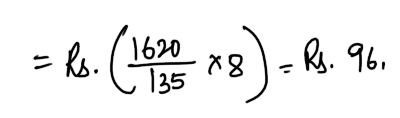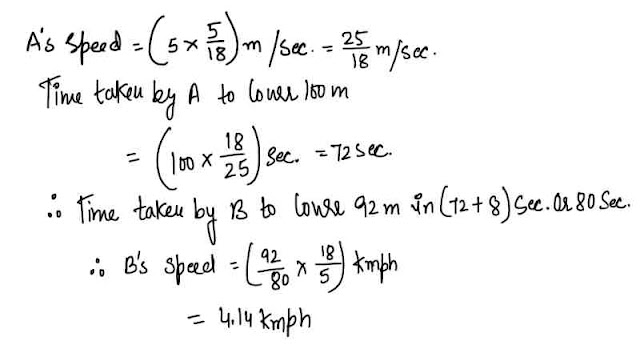# Mixed Aptitude Quiz for SBI Clerk Mains (Part - 14)#### Ques 1.

Two pipes A and B Together can fill a cistern in 4 hours.Had they been opened separately, then B would have taken 6 hours more than A to fill the cistern. How much time will be taken by A to fill the cistern separately ?
(a) 1 hour
(b) 2 hour
(c) 6 hour
(d) 8 hour
Ans 1. suppose pipe A alone takes x hours to fill the cistern
. . B alone takes ( x+ 6) hours to fill the  cistern.#### Ques 2.

In the wheat sold by a grocer, 10% of the wheat was of inferior quality.In 150 kg of wheat , what mixed such that the quantity of the inferior quality wheat will be 5% ?
(a) 150 kg
(b) 135 kg
(c) 50 kg
(d) 85 kg
Ans 2. In 150 kg of wheat , there is 135 kg of  good quality wheat and 15kg  of inferior  quality wheat.
Let x  kg of good quality wheat be mixed  in 135 kg good quality wheat so that the total quantity of good quality wheat becomes 95% of the total wheat.
i.e. , 95% of ( 150 + x).
. . 135 + x   = 95% of ( 150 + x)
i.e. x  = 150

#### Ques 3.

The least number which when divided by 5,6,7 and 8 leaves a remainder 3, but when divided by of the stream ?
(a) 1677
(b) 1683
(c) 2523
(d) 3363
Ans 3. L.C.M of 5,6,7,8 = 840 k + 3
Least value of K for which ( 840 k + 3) is  divisible  by 9 is k = 2
. . Required number = ( 840 x 2 + 3)  = 1683

#### Ques 4.

In a box carrying one dozen of oranges, one-third have become bad. If 3 oranges are taken out from the box at random, what is the probability that at least one orange out of three oranges picked up is good ?
(a) 1/55
(b) 54/55
(c) 45/55
(d) 3/55
Ans 4.  Total number of oranges = 12
The number of bad oranges = 12 x 1/3 = 40
. . number of good oranges = 12 -4 = 8.
Now, the total number of ways of selecting 3 oranges from the box = 12c3.
The Total number of ways  of selecting  3 bad  oranges  out of 4 bad oranges = 4C3
Hence, the probability  of selecting  all the three bad oranges ( no good oranges )
= 4C3/12C3 = 1/55
Therefore, the probability of selecting at least  one good orange = 1 - 1/55 = 54/55\

#### Ques 5.

A,B,C started a business with their investments in the ratio 1: 3 : 5. After 4 months , A invested the game amount as before and B as well as C withdrew half of their investment . The ratio of their profits at the end of the year is
(a) 5 : 6 : 10
(b) 6 : 5 : 10
(c) 10 : 5 : 6
(d) 4 : 3 : 5
Ans 5. Let their initial investment be x, 3x, 5x Then
A : B : C  = x x 4 + 2 x  x 8  : 3x  x 4  + 3x/z x 8 : 5x x 4 + 5x/2 x 8
= 20x : 24x : 40x = 5 : 6 : 10.

#### Ques 6.

Total salary of three persons A, B and C is Rs.144000. They spend 80%, 85% and 75% respectively.If their savings are as 8 : 9 : 20, then the salary of C is
(a) Rs 32,000
(b) Rs 40,000
(c) Rs 48,000
(d) Rs 64,000
Ans 6. Let the salaries  of A,B  and C be x , y , z  respectively
. .#### Ques 7.

By investing Rs . 1620 in 8% stock, Gopal earns Rs. 135. The stock is then quoted at
(a) Rs 106
(b) Rs 96
(c) Rs 80
(d) Rs 108
Ans 7.  To earn Rs. 135, investment = Rs  1620
To earn Rs 8, investment. .  Mraket  value of rs. 100 stock = Rs 96.

#### Ques 8.

A and B take part ina 100 m race. A runs at 5 km per hour. A gives B a start of 8 m and still beats him by 8 seconds. The speed of B is
(a) 5 .15 kmph
(b) 4.14 Kmph
(c) 4.25 kmph
(d) 4 .4 Kmph
Ans 8.#### Ques 9.

The first republic day of India was celebrated on 26th January , 1950. It was
(a) Monday
(b) Tuesday
(c) Thursday
(d) Friday
Ans 9.  26th January , 1950 means.
= ( 1949 years+ period from Ist January  to 26th January)
Number of odd days in ( 1600 + 300) years
= ( 0 + 1) = 1
49 years = ( 12  leap years + 37 ordinary years) = ( 24 + 37) odd days = 61 odd days = 5 odd days
26 days  of January  have 5 odd days.
, . Total number of odd days = ( 1 + 5 + 5)
4 odd days
The desired day was = Thursday.

#### Ques 10.

3 men and 4 boys can earn Rs.756 in 7 days. 11 men and 13 boys can earn Rs. 3008 in 8 days. In what time will 7 men with 9 boys earn Rs. 2480 ?
(a) 13 days
(b) 10 days
(c) 15 days
(d) 12 days
Ans 10.Let at man's  one day wage be M  and  a boy's  one day  wage be B.
. . 3M + 4B= 756/7 = 108
and 11M  + 13B = 3008/8 = 376
solving the two equations , we get wage of a man  for a days work = rs 20& wage of a boy a day's  work = Rs 12.
. . Combined wages  of 7 men  + 9 boys for a day ;s work = 140 + 108 = Rs. 248
No. of days required = 2480/248 = 10 days.